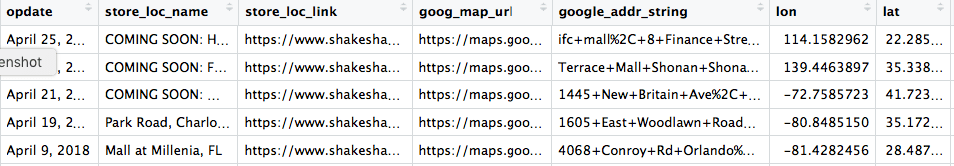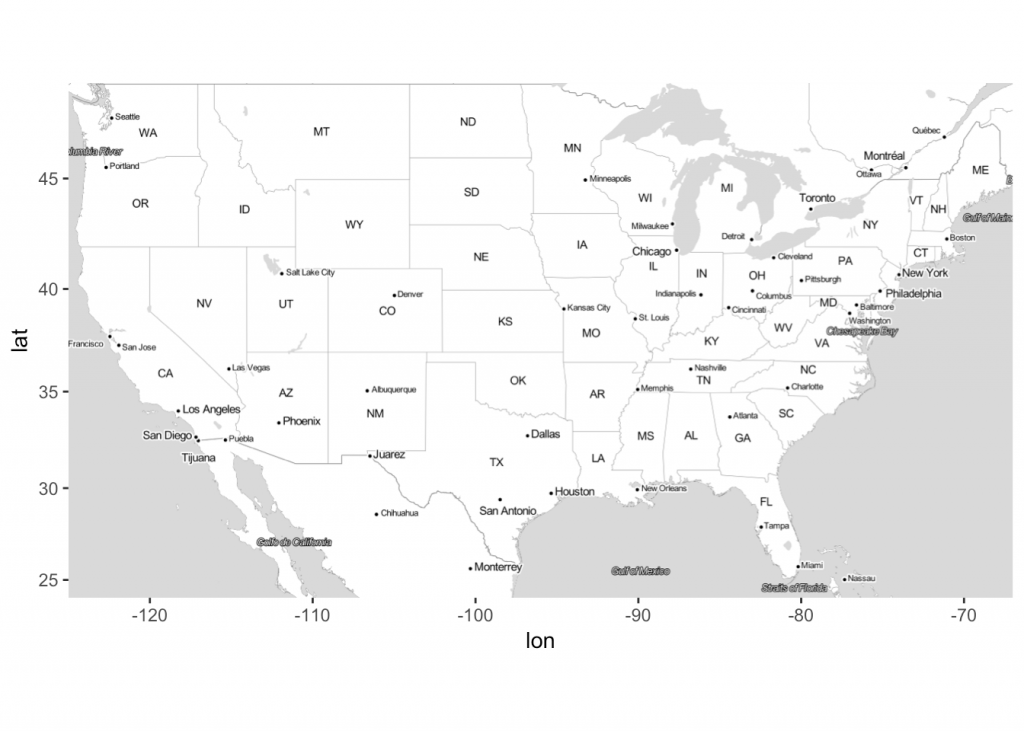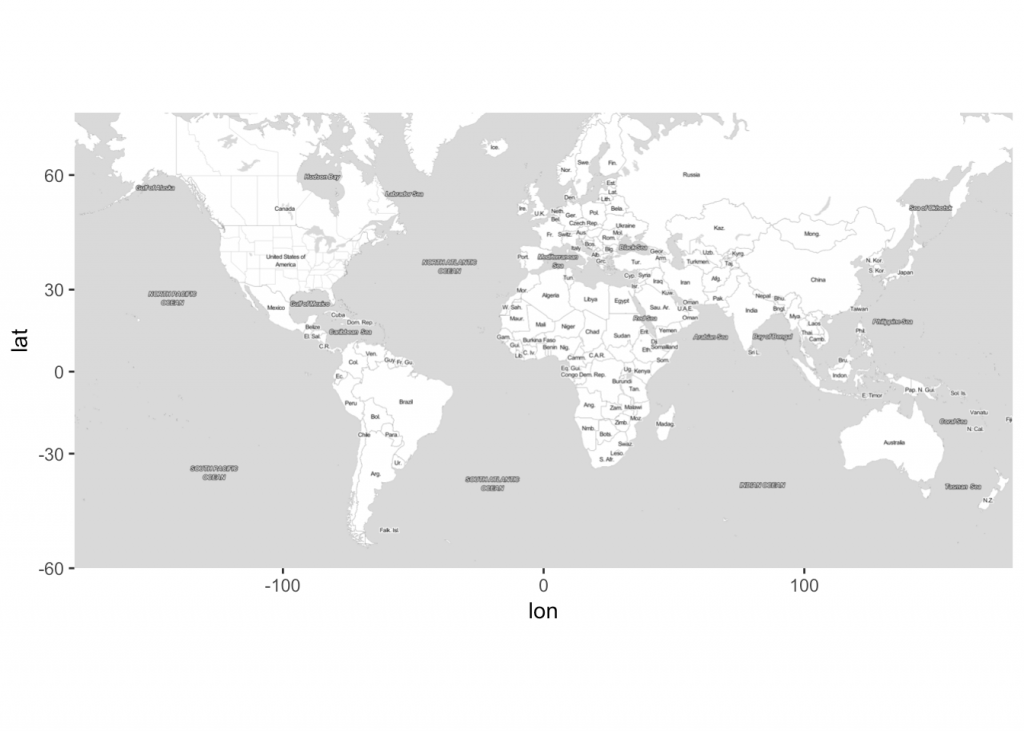Want to share your content on R-bloggers? click here if you have a blog, or here if you don't.

I had heard that the lines to get some food at Shake Shack are long. So when I saw a new location opening in downtown LA, I wondered how many locations does it have and how fast are they spreading across the US. The answers surprised me. Using R and previous code, I created a few maps:Read on to learn how I got the data and plotted them.

First, let’s load our favourite libraries.

```library(rvest)
library(tidyverse)
library(scales)
library(ggmap)```

## Figure out locations

On its site, Shake Shack fortunately has all the locations and opening dates, going back to April 23, 2012. The archive pages run from 1 to 20 with this URL structure:

`https://www.shakeshack.com/location/page/`

Using SelectorGadget, I figured out the XPath and CSS code to find the opening date, location name, and location page link. Then, I wrote a function to retrieve these values from a given archive page.

```get_locations <- function(url) {
nodes <- page_html %>%
html_nodes(xpath = '//*[contains(concat( " ", @class, " " ), concat( " ", "span4", " " ))]')

data.frame(opdate = html_nodes(x = nodes,
xpath = '//*[contains(concat( " ", @class, " " ), concat( " ", "date", " " ))]') %>%
html_text(trim = TRUE),
store_loc_name = html_nodes(x = nodes,
css = 'h2') %>%
html_text(trim = TRUE),
css = 'h2 a') %>%
html_attr("href"),
stringsAsFactors = FALSE)
}```

I applied this function to retrieve all location opening dates, names, and individual location urls:

```all_loc_pages <- paste0("https://www.shakeshack.com/location/page/", 1:20, "/")

all_locations <- do.call(rbind, lapply(all_loc_pages, get_locations))```

## Find addresses of all locations

If you visit an individual location’s page, such as this Tokyo Dome page, you will see that often the exact address is not listed, or if it is, you can’t directly geocode it. But, luckily, there’s a Google Map right below the location. I thought, they must be passing some parameters to Google Maps API. I spend a good amount of time, but couldn’t figure out how they were getting the map. And. Then. I found out that the text “CLICK MAP FOR DIRECTIONS” block had a valid address as part of the hyperlink!!I wrote another simple function to get the addresses from the given URL:

```get_loc_cords <- function(loc_url) {
data.frame(loc_url = loc_url,
goog_map_url =  location_html %>%
html_attr("href"),
stringsAsFactors = FALSE)
}

Then I joined the location name with the address data frame:

`all_locations <- left_join(all_locations, location_google_maps_address, by = c("store_loc_link" = "loc_url"))`

Using the fantastic ggmap library and mutate_geocode function, I geocoded all the addresses:

```all_locations <- all_locations %>%

Here’s what the data frame looks like now:### Tip

You may want to create a Google developer key for mass geocoding. Since the mutate_geocode function is used by many people, sometimes you may not get all the addresses geocoded. Use register_google(key = , account_type = 'premium', day_limit = 100000) function to register your key with ggmap functions.

## Data manipulation

Now that we have all the geographical coordinates, we just need to do some clean-up to get the data ready for plotting.

First, get the date field in order and add opening month and year columns:

```all_locations <- all_locations %>%
mutate(open_date = as.Date(opdate, "%B %d, %Y"),
open_month = lubridate::month(open_date),
open_year = lubridate::year(open_date))```

Second, get the cumulative count of store openings:

```ss_op_data_smry <- all_locations %>%
count(open_date) %>%
ungroup() %>%
arrange(open_date) %>%
mutate(cumm_n = cumsum(n))```

Third, join the summary back to the locations data frame:

```all_locations_smry <- inner_join(all_locations, ss_op_data_smry,
by = c("open_date" = "open_date"))```

Using the ggmap library, I got the US map and a world map:

```us_map <- get_stamenmap(c(left = -125, bottom = 24, right = -67, top = 49), zoom = 5, maptype = "toner-lite")
ggmap(us_map)``````world_map <- get_stamenmap(bbox = c(left = -180, bottom = -60, right = 179.9999, top = 70), zoom = 3, maptype = "toner-lite")
ggmap(world_map)```## Create functions to plot each location

Repurposing my code from the Walmart spread across the US, I wrote a similar function to plot locations with two different sizes: big, if the locations opened during the mapped month, and small, if the locations opened before the mapped month. I did so that we could notice the new locations.

```my_us_plot <- function(df, plotdate, mapid){
g <- ggmap(us_map, darken = c("0.8", "black"), extent = "device")
old_df <- filter(df, open_date < plotdate)
new_df <- filter(df, open_date == plotdate)
# old locations
g <- g + geom_point(data = old_df, aes(x = lon, y = lat), size = 5, color = "dodgerblue", alpha = 0.4)
# new locations
g <- g + geom_point(data = new_df, aes(x = lon, y = lat), size = 8, color = "dodgerblue", alpha = 0.4)
g <- g + theme(axis.ticks = element_blank(), axis.title = element_blank(), axis.text = element_blank(), plot.title = element_blank(), panel.background = element_rect(fill = "grey20"), plot.background = element_rect(fill = "grey20"))
g <- g + annotate("text", x = -77, y = 33, label = "MONTH/YEAR:", color = "white", size = rel(5), hjust = 0)
g <- g + annotate("text", x = -77, y = 32, label = paste0(toupper(month.name[unique(new_df\$open_month)]), "/", unique(new_df\$open_year)), color = "white", size = rel(6), fontface = 2, hjust = 0)
g <- g + annotate("text", x = -77, y = 31, label = "STORE COUNT:", color = "white", size = rel(5), hjust = 0)
g <- g + annotate("text", x = -77, y = 30, label = comma(unique(new_df\$cumm_n)), color = "white", size = rel(6), fontface = 2, hjust = 0)
ggsave(filename = filename, plot = g, width = 13, height = 7, dpi = 120, type = "cairo-png")
}```

I modified this function to map the world:

```my_world_plot <- function(df, plotdate, mapid){
g <- ggmap(world_map, darken = c("0.8", "black"), extent = "device")
old_df <- filter(df, open_date < plotdate)
new_df <- filter(df, open_date == plotdate)
g <- g + geom_point(data = old_df, aes(x = lon, y = lat), size = 5, color = "dodgerblue", alpha = 0.4)
g <- g + geom_point(data = new_df, aes(x = lon, y = lat), size = 8, color = "dodgerblue", alpha = 0.4)
g <- g + theme(axis.ticks = element_blank(), axis.title = element_blank(), axis.text = element_blank(), plot.title = element_blank(), panel.background = element_rect(fill = "grey20"))
g <- g + annotate("text", x = -130, y = 0, label = "MONTH/YEAR:", color = "white", size = rel(5), hjust = 0)
g <- g + annotate("text", x = -130, y = -10, label = paste0(toupper(month.name[unique(new_df\$open_month)]), "/", unique(new_df\$open_year)), color = "white", size = rel(6), fontface = 2, hjust = 0)
g <- g + annotate("text", x = -130, y = -20, label = "STORE COUNT:", color = "white", size = rel(5), hjust = 0)
g <- g + annotate("text", x = -130, y = -30, label = comma(unique(new_df\$cumm_n)), color = "white", size = rel(6), fontface = 2, hjust = 0)
ggsave(filename = filename, plot = g, width = 12, height = 6, dpi = 150, type = "cairo-png")
}```

## Create maps

Now, the exciting part: create month-by-month maps.

US maps:

```all_locations_smry %>%
mutate(mapid = group_indices_(all_locations_smry, .dots = 'open_date')) %>%
group_by(open_date) %>%
do(pl = my_us_plot(all_locations_smry, unique(.\$open_date), unique(.\$mapid)))```

World maps:

```all_locations_smry %>%
mutate(mapid = group_indices_(all_locations_smry, .dots = 'open_date')) %>%
group_by(open_date) %>%
do(pl = my_world_plot(all_locations_smry, unique(.\$open_date), unique(.\$mapid)))```

## Create a movie

Using ffmpeg, we can put all the images together to create a movie:

```# works on a mac
makemovie_cmd <- paste0("ffmpeg -framerate 8 -y -pattern_type glob -i '", paste0(getwd(), "/maps/"), "*.png'", " -c:v libx264 -pix_fmt yuv420p '", paste0(getwd(), "/maps/"), "movie.mp4'")
system(makemovie_cmd)```

We can use the convert function to create a gif:

```# https://askubuntu.com/a/43767
makegif_cmd <- paste0("convert   -delay 8   -loop 0 ", paste0(getwd(), "/maps/"), "*.png ", "animated.gif") # loop 0 for forever looping
system(makegif_cmd)```

That’s it! We get nice looking videos showing location openings by each month. I was surprised to see how fast the company is opening the locations as well as how many locations it has in Asia!

## Post hoc

Using the ggimage library, I tried creating the maps using Shake Shack’s burger icon, but they didn’t turn out as good:

```my_us_icon_plot <- function(df, plotdate, mapid){
g <- ggmap(us_map, darken = c("0.8", "black"))
old_df <- filter(df, open_date < plotdate)
new_df <- filter(df, open_date == plotdate)
g <- g + geom_image(data = old_df, aes(x = lon, y = lat), image = "ss-app-logo.png", by = "height", size = 0.03, alpha = 0.4)
g <- g + geom_image(data = new_df, aes(x = lon, y = lat), image = "ss-app-logo.png", by = "height", size = 0.07, alpha = 0.4)
g <- g + theme(axis.ticks = element_blank(), axis.title = element_blank(), axis.text = element_blank(), plot.title = element_blank())
g <- g + annotate("text", x = -77, y = 33, label = "MONTH/YEAR:", color = "white", size = rel(5), hjust = 0)
g <- g + annotate("text", x = -77, y = 32, label = paste0(toupper(month.name[unique(new_df\$open_month)]), "/", unique(new_df\$open_year)), color = "white", size = rel(6), fontface = 2, hjust = 0)
g <- g + annotate("text", x = -77, y = 31, label = "STORE COUNT:", color = "white", size = rel(5), hjust = 0)
g <- g + annotate("text", x = -77, y = 30, label = comma(unique(new_df\$cumm_n)), color = "white", size = rel(6), fontface = 2, hjust = 0)
ggsave(filename = filename, plot = g, width = 13, height = 7, dpi = 150, type = "cairo-png")
}```

## Fun mapsWhat do you think? How else would you visualize these data points?

The post Do know Shake Shack’s locations outside of the US? You’d be surprised appeared first on nandeshwar.info.